Smartick is a fun way to learn math!Nov26

# Perimeter: What Is It and How to Find It for Any Polygon

Today we are going to learn to calculate perimeters of geometric figures. But first, let’s start with the definition.

Index

## What is a Perimeter?

A perimeter is what we call the length of the outline of a geometric figure.

Therefore, the perimeter is a measurement of length, so it will come in centimeters, inches, and feet, generally in linear units.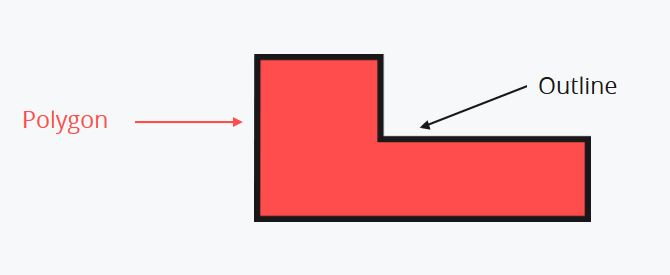You can review the most common types of polygons here or take a look at the following video.

## Video: Learn How to Calculate Perimeters

The story of Eva and the garden at her school can help you better understand the concept of perimeter. This is a video of one of our interactive tutorials. It is no longer interactive but you have the advantage of being able to watch it as many times as necessary and sharing it with others. If you would like to access the real interactive tutorials, register with Smartick, the online math learning method for children ages 4 to 14.

## How to Find a Perimeter

Let’s take a look at the first strategy for calculating perimeters. It does not matter how many sides the polygon has.

The perimeter of a geometric figure can always be calculated by adding the length of each of their sides.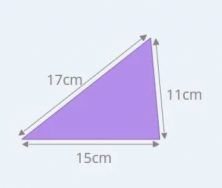In order to the calculate the perimeter you need to add the length of its sides: 17cm + 15cm + 11cm = 43cm

You can use this strategy to calculate the perimeter of any polygon.

## How to Find The Perimeter of Polygons with Examples

Now that you know what a perimeter is and how to calculate it for any polygon, we are going to see how to calculate the perimeter for each of the following geometric figures:

### How to Find The Perimeter of a Square

The special characteristic of a square is that it has four equal sides. We can take advantage of this by simplifying our calculations.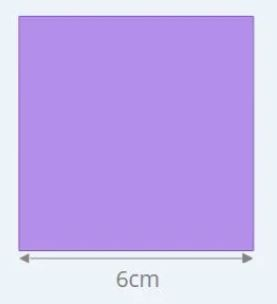You can calculate the perimeter of this square by adding together the lengths of the four sides.

Perimeter = 6cm + 6cm + 6cm + 6cm = 24cm

Since the four sides are equal, multiplying the length of one side by four will give you the same result.

Perimeter = 4 x 6cm = 24cm

The perimeter of a square = 4 x length of one side

### How to Find the Perimeter of a Rectangle

In every rectangle, the opposite sides are equal to one another. It has sides that are equal, two by two.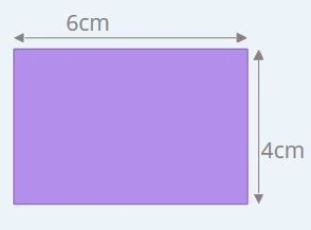In order to calculate the perimeter of the rectangle in the example, you can add the length of its sides, two 6cm sides and two 4 cm sides.

Perimeter = 6cm + 4cm + 6cm + 4cm = 20cm

You can multiply the sum of the base and height by two and obtain the same result because all rectangles repeat the length of their sides two times.

Perimeter = 2x (6cm + 4cm) = 20cm

So, you have an approach for any rectangle.

The perimeter of a rectangle = 2 x (base + height)

### How to Find the Perimeter of Triangles

#### Equilateral Triangles

The same as with squares, the sides of an equilateral triangle are equal. They all measure the same length.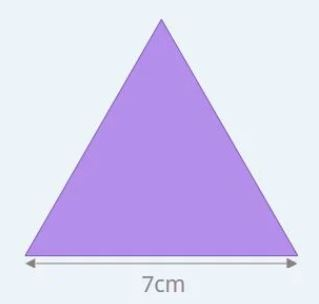Each side measures 7cm and you can calculate the length of its outline in the following way.

Perimeter = 7cm + 7cm + 7cm = 21cm

Or, there is an easier way. Since the three sides are the same, you can multiply one side by 3 and the result does not change.

Perimeter = 3 x 7cm = 21cm

And this helps you with any equilateral triangle.

The perimeter of an equilateral triangle = 3 x length of one side

#### Isosceles Triangles

Isosceles triangles have two equal sides and one that is different.

To refresh your memory about the different types of triangles, take a look at this post on geometric shapes.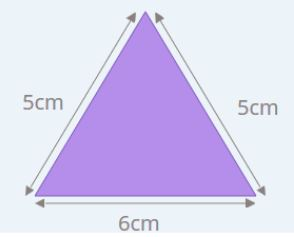Given that two sides are equal and one is different, we just need to multiply the length of the repeated side by two and then add the length of the side that is different.

Perimeter = 5cm x 2 + 6cm = 16cm

So, for any isosceles triangle:

The perimeter of an isosceles triangle = length of repeated side x 2 + length of different side

### How to Find the Perimeter of a Rhombus

A rhombus has four equal sides. But not all of its angles are equal, only the angles that are opposite one another.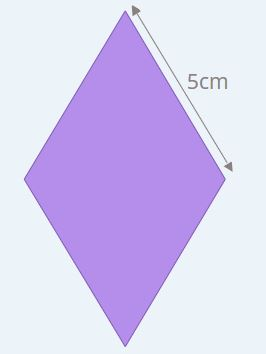Since the four sides are equal, we can multiply the length of one side by four in order to get the perimeter.

Perimeter = 4 x 5cm = 20cm

This rule is the same for squares because they also have four equal sides.

The perimeter of a rhombus = 4 x length of one side

### How to Find the Perimeter of a Trapezoid

#### Isosceles Trapezoid

The isosceles trapezoids have a special way of calculating their perimeter. They have two opposite sides that are equal and the other two sides, known as the bases (top and bottom), are parallel but different lengths.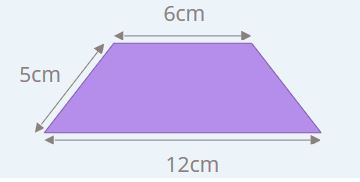In this case, we need to multiply the length of one of the opposite sides by two and add the lengths of the two bases.

Perimeter = 5cm x 2 + 12cm + 6cm = 28cm

To calculate the perimeter for any isosceles trapezoid:

The perimeter of an isoceles trapezoid = length of opposite side x 2 + length of top base + length of bottom base

### How to Find the Perimeter of Stepped Polygons

Stepped polygons have a very peculiar characteristic. The sum of the lengths of the sides that are parallel to the base measure the same as the length of the base. And the same goes for the sum of the lengths of the sides parallel to the height, which measure the same length as the height.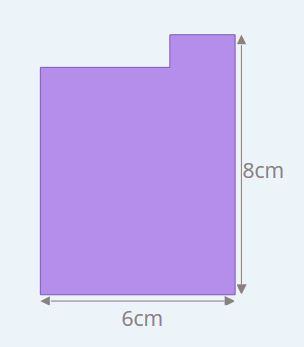So, to calculate the perimeter of any stepped polygon we can use the same formula that we use for rectangles because we can approach the sum of the lengths of the horizontal and vertical sides as if they were equal to the length of the base and height. It is as if the lengths of the base and height were repeated.

Perimeter = 2x (6cm + 8cm) = 28cm

The rule works for any stepped polygon of this type:

The perimeter of a scalene polygon = 2 x (base + height)

### How to Find the Perimeter of Any Regular Polygon

The defining feature of regular polygons is that all of their sides are the same length.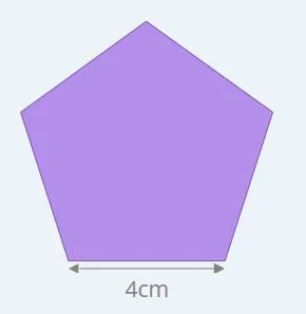Since a pentagon has five equal sides, to find its perimeter you multiply the length of one side by five.

The perimeter of a pentagon = 5 x length of one side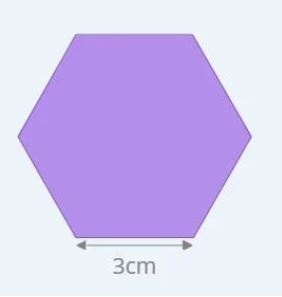And with a hexagon, which has six equal sides, you can multiply one side by six.

The perimeter of a hexagon = 6 x length of one side

From these rules, we are able to extract a rule to calculate the perimeter of any regular polygon in a simple way.

Multiply the number of sides of the polygon by the length of one of the sides.

The perimeter of a regular polygon = number of sides x length of one side

### How to Calculate the Perimeter of a Circle

To calculate the perimeter of a circle, the first thing you need to know is that the perimeter of a circle is equal to the length of its circumference.

To calculate the length of a circumference you have to multiply the diameter of the circumference by the Pi:

• The perimeter of a circle is equal to Pi times the diameter (d): Perimeter of a circle = π x d
• You can also multiply 2 by Pi by the radius (r): Perimeter of a circle = 2.π x r, this is because the diameter is double the radius, always.

The formulas we have just seen use number Pi, whose symbol is π, and comes from the number representing the ratio between the length of a circumference and its diameter (d), π=Perimeter of a circle/d. It has infinite decimal places, but in school, it is usually rounded to the hundredths: π ≃ 3.14.